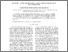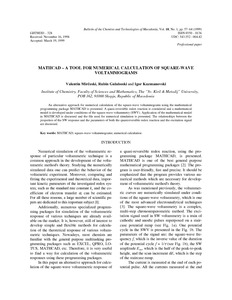# MATHCAD – a Tool for Numerical Calculation of Square-Wave Voltammograms

Mirceski, Valentin and Gulaboski, Rubin (1999) MATHCAD – a Tool for Numerical Calculation of Square-Wave Voltammograms. Bulletin of the Chemists and Technologists of Macedonia, 18 (1). pp. 57-64. ISSN 0350 – 0136Preview Text __ugd.edu.mk_private_UserFiles_rubin.gulaboski_Desktop_GULABOSKI-MY pdf PUBLICATIONS_MATHCAD PAPER.pdf Download (202kB) | Preview

## Abstract

An alternative approach for numerical calculation of the square-wave voltammograms using the mathematical
programming package MATHCAD is presented. A quasi-reversible redox reaction is considered and a mathematical
model is developed under conditions of the square-wave voltammetry (SWV). Application of the mathematical model
in MATHCAD is discussed and the file used for numerical simulation is presented. The relationships between the
properties of the SW response and the parameters of both the quasireversible redox reaction and the excitation signal
are discussed.

Item Type: Article Natural sciences > Chemical sciencesNatural sciences > MatematicsNatural sciences > Physical sciences Faculty of Agriculture Rubin Gulaboski 31 Oct 2012 23:09 15 Nov 2012 10:22 https://eprints.ugd.edu.mk/id/eprint/209View Item